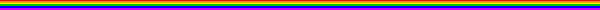# Derivative Module

### Lisa Denise MurphyThese twelve pages take the students through about a week's worth of in-class activities and homework. By the end of the week, they should understand how the distance and velocity graphs represent distance, direction of motion, speed, change in speed, and velocity. They should be able to interpret distance and velocity graphs, and discuss their conclusions in both qualitative and quantitative terms.

The word "derivative" is not introduced in these pages. When I figure out how to convert Mathematica files into web documents, I will add the pages introducing the difference quotient and the derivative.1. Meeting the motion sensor, and exploring the way the distance graph represents distance.

2. Exploring the way the distance graph represents direction of motion.

3. Exploring the way the distance graph represents speed of motion.

4. Exploring the way the distance graph represents changes in speed.

5. Quantifying speed of motion based on interpreting the distance graph.

6. Introducing velocity as a vector quantity (with both magnitude and direction).

7. Introducing the velocity graph, and exploring the way the distance and velocity graphs represent distance.

8. Exploring the way the velocity graph represents direction of motion.

9. Exploring the way the velocity graph represents speed of motion.

10. Exploring the way the velocity graph represents changes in speed.

11. Quantifying speed of motion based on interpreting the velocity graph.

12. Relating the distance graph to the velocity graph in both qualitative and quantitative ways.Back to Lisa's Derivative Project page.

Back to Lisa's Academic Activities page.

Back to Lisa's personal home page, Over the Rainbow.

This page last revised February 4, 1999.